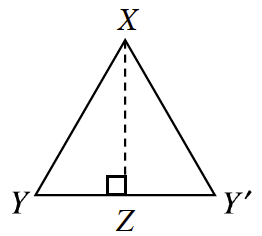### Home > INT1 > Chapter 7 > Lesson 7.1.4 > Problem7-47

7-47.$ΔXYZ$ was reflected across $\overline{XZ}$, as shown at right.

1. How can you justify that the points $Y$$Z$, and $Y'$ all lie on a straight line?

$m∠YZX=90º$

2. What is the relationship between $ΔXYZ$ and $ΔXY'Z$? Why?

Remember that reflections do not change the size or shape of a figure.

3. Read the Math Notes box for this lesson. Then make all the statements you can about the corresponding parts of these two triangles.

Altogether, you should be able to make six statements.
Be sure to make them for all corresponding angles and sides.